# AP Chemistry : Solubility

## Example Questions

← Previous 1 3

### Example Question #1 : Solubility

What is the formula for the dissociation of iron (II) phosphate?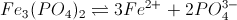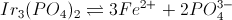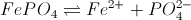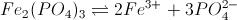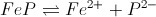Explanation:

Iron (II) has a positive two charge: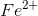.

Phosphate has a negative three charge: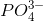.

The initial compound must be constructed to cancel these charges. The dissociation is: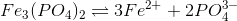.

### Example Question #2 : Solubility

Pb(OH)2 has a Ksp of  1.43 * 10-20.

What is its solubility?

1.20 * 10-10M

1.53 * 10-7M

2.43 * 10-7M

8.42 * 10-12M

1.53 * 10-7M

Explanation:

Lead (II) hydroxide dissociates according to this reaction: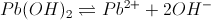. Solubility is equal to the number of moles that will dissociate at equilibrium, and can be found using this reaction and the value of Ksp.

Ksp = [Pb2+][OH-]2

If [Pb2+] = x, then [OH-] = 2x. They exist in a 1:2 ratio.

Ksp = 1.43 * 10-20 = x(2x)2 = 4x3

x = 1.53 x 10-7M

### Example Question #1 : Solubility

Which of the following factors will generally lead a solute to dissolve more easily into a solution (i.e. increase solubility)?

None of these choices will increase solubility

Decreasing the temperature of the solution

The common ion effect

Increasing the temperature of the solution

Increasing the temperature of the solution

Explanation:

Increasing the temperature of a solution will generally increase its solubility, while decreasing temperature will have the opposite effect. The common ion effect refers to the phenomenon when two compunds in a solution dissociate to produce at least one similar ion, and will make it harder for additional amounts of that ion in the solution to dissociate, decreasing solubility.

### Example Question #1 : Solubility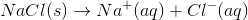If the Ksp of the above dissociation is found to be 2.70 * 10-4, what is the solubility of NaCl?

1.44 * 10-2M

7.29 * 10-8M

1.89 * 10-2M

1.64 * 10-2M

1.64 * 10-2M

Explanation:

First, set up a balanced reaction and enter X for the amount of each solute that is going to be dissolved. As NaCl dissociates, each ion amount will increase by some amount, X.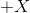Then set up the equation for the value of Ksp and enter in the value of X for each solute. Use this equation to solve for X, the solubility (in mol/L). Remember that NaCl si not included in the equation, as it is a pure solid.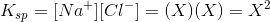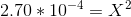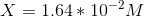### Example Question #5 : Solubility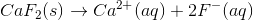If the solubility of CaF2 in the dissociation reaction above was determined to be 1.54 * 10-3M, what is the Ksp value for CaF2?

1.42 * 10-6

1.98 * 10-8

2.24 * 10-6

1.46 * 10-8

1.46 * 10-8

Explanation:

Use the balanced reaction of the dissociation to assign values of X for the solubility of each ion. As CaF2 dissociates, each ion amount will increase by some amount; calcium will increase by X and flouride will increase by 2X.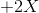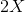Then plug in the equation to solve Ksp, using the X values assigned for each solute. Use the given value for solubility to find Ksp. Remember that NaCl si not included in the equation, as it is a pure solid.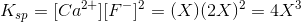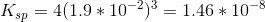### Example Question #6 : Solubility

Consider the following balanced equation for the solubility of barium hydroxide in an aqueous solution.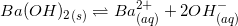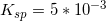What is the solubility of barium hydroxide?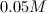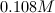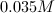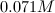Explanation:

In order to solve for the solubility of barium hydroxide, we need to establish an ICE table for the reaction.

I. Initially, there are no barium ions or hydroxide ions in the solution, so we can call their concentrations zero at the beginning of the reaction.

C. For every molecule of dissolved barium hydroxide, there will be one ion of barium and two ions of hydroxide. As a result, the increases in each ion can be designated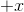and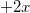, respectively.

E. Finally, we plug these values into the equilibrium expression and set it equal to the solubility product constant.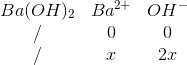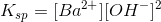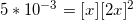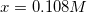### Example Question #1 : Solubility

Four different insoluble salts are added to an aqueous solution in equal amounts. Assume that the salts do not interact with one another in any way. The following list shows the salts and their solubility product constants.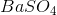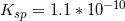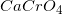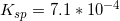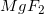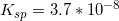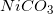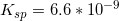Which of the following ions will have the highest concentration in the solution?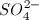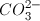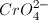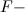Explanation:

The solubility product constants help us envision which ions will have the largest concentrations after dissolving to equilibrium.has the largest solubility product constant, meaning it will dissolve the most out of the four options.

Upon the dissolving of, twice the number of fluoride ions will be released as magnesium ions; however, the solubility constant for magnesium fluoride is so much smaller, compared to the solubility constant of, that it will not become the ion with the largest concentration.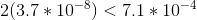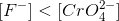### Example Question #8 : Solubility

Consider the following balanced equation for the dissociation of barium hydroxide in an aqueous solution.A 1M solution of barium nitrate is added to the solution. What is the solubility of barium hydroxide after this addition?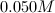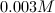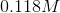Explanation:

Before we solve for the solubility, remember Le Chatelier's principle. Barium nitrate is a soluble salt that will completely dissolve in solution. This means that there will be a one molar concentration of barium ions before the salt dissolves. Since we have added to the products side of the reaction, we can predict that the equilibrium will be shifted to the left, and the barium hydroxide will be less soluble. This change in equilibrium is referred to as the common ion effect.

We will use an ICE table to determine the solubility of the salt.

I. Before the salt begins to dissolve, there is a one molar concentration of barium ions (from the barium nitrate) with no hydroxide ions in the solution.

C. Since every dissolved molecule of barium hydroxide will yield one barium ion and two hydroxide ions, the unknown increases in each ion can be designated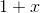andrespectively. Notice how the barium ion increase will be added to the previously added one molar concentration in the solution.

E. Now, we set the solubility expression equal to the solubility product constant.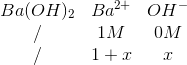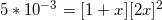Since 1 is such a larger number than the value we will get for, we can consider the value in the barium ion expression to be negligible. This allows us to simplify the equation to the following setup.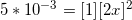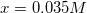The solubility of barium hydroxide without the common ion effect is 0.108M. Notice how the solubility for the salt has decreased, as was predicted in the beginning by Le Chatelier's principle.

### Example Question #71 : Solutions

A chemist has a fully saturated solution of chemical X, with solid X resting on the bottom. If she adds a chemical that will react with the aqueous X, what will happen to the solid?

It will start to dissolve into the solution

More solid will precipitate out of solution

The solid will change color

Nothing will change

A layer of sediment will cover the solid

It will start to dissolve into the solution

Explanation:

A fully saturated solution is at equilibrium between the solid and aqueous states, but it is a dynamic equilibrium. As the reacting chemical reacts with aqueous X, more and more of the solid will dissolve into solution.

### Example Question #1 : Solubility

The partial pressure of an arbitrary gas,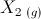, in an enclosed chanber with a pool of water is 7.2atm at 298K.

The Henry's Law constant foris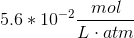.

What is the solubility of?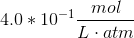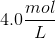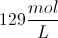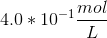Explanation:

Henry's law can be expressed: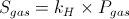Where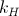is the Henry's Law constant, and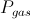is the partial pressure of the gas above the solution. We can substitute our givens into this equation: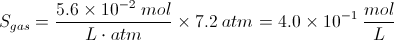← Previous 1 3